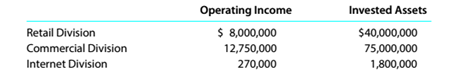Chapter 14, Problem 14.10E

Chapter
Section
Textbook Problem

Return on investment The operating income and the amount of invested assets in each division of Oe Industries are as follows:a. Compute the return on investment for each division. b. Which division is the most profitable per dollar invested?

To determine

Concept Introduction:

Return on investment: In this we divide the total return or profit with the investment into the assets of organization. It is the best way of calculating the % of return in the business.

Requirement-1:

To Identify:

Find out the return on investment ratio.

Explanation

By following we calculate the Return on investment:

 Year Retail Commercial Internet Investment 4000000...
To determine

Concept Introduction:

Return on investment: In this we divide the total return or profit with the investment into the assets of organization. It is the best way of calculating the % of return in the business.

Requirement-2:

To Identify:

Find out the Most Profitable division of the organization?

Still sussing out bartleby?

Check out a sample textbook solution.

See a sample solution

The Solution to Your Study Problems

Bartleby provides explanations to thousands of textbook problems written by our experts, many with advanced degrees!

Get Started

Find more solutions based on key concepts